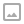## Dinic学习笔记

·  ✏️ About  1335 words  ·  ☕ 3 mins read · 👀... views

Dinic算法是一种用于网络流中最大流的增广路算法，其时间复杂度为$O(n^2 \times m)$，但大多数情况下会远远优于此时间复杂度。

## 基本概念

• 容量： ${capacity}(e)$ 表示一条有向边 $e(u,v)$ 的最大允许的流量。

• 流量： ${flow}(e)$ 表示一条有向边 $e(u,v)$ 总容量中已被占用的流量。

• 剩余容量（残量）：即 $capacity(e)−flow(e)$，表示当前时刻某条有向边 $e(u,v)$ 总流量中未被占用的部分。

• 反向边：原图中每一条有向边在残量网络中都有对应的反向边，反向边的容量为$0$，容量的变化与原边相反；『反向边』的概念是相对的，即一条边的反向边的反向边是它本身。

• 残量网络：在原图的基础之上，添加每条边对应的反向边，并储存每条边的当前流量。残量网络会在算法进行的过程中被修改。

• 增广路（augmenting path）：残量网络中从源点到汇点的一条路径，增广路上所有边中最小的剩余容量为增广流量。

• 增广（augmenting）：在残量网络中寻找一条增广路，并将增广路上所有边的流量加上增广流量的过程。

• 层次： $level(u)$ 表示节点 $u$ 在层次图中与源点的距离。

• 层次图：在原残量网络中按照每个节点的层次来分层，只保留相邻两层的节点的图，满载（即流量等于容量）的边不存在于层次图中。

## 思路

1. 建立以出发点为源点的层次图（即源点到各店的距离）
2. 在层次图&残量网络中寻找增广路，并增广流量
3. 重复2直到找不到增广路
4. 重复123直到不存在层次图


## 代码

Luogu P3376为例

  1 2 3 4 5 6 7 8 9 10 11 12 13 14 15 16 17 18 19 20 21 22 23 24 25 26 27 28 29 30 31 32 33 34 35 36 37 38 39 40 41 42 43 44 45 46 47 48 49 50 51 52 53 54 55 56 57 58 59 60 61 62 63 64 65 66 67 68 69 70 71 72 73 74 75 76 77 78 79 80 81 82 83 84  #include #include #include #include using namespace std; struct Edge{ int from,to,flow,cap; int next; }edge; int fir,dis,cur; int n,m,s,t,tot = 2;//tot从2开始是最舒服的，既可以直接异或，后面的终止条件也不用想来想去。 bool bfs(){ queue q; memset(dis,0,sizeof(dis)); memcpy(cur,fir,sizeof(fir));//清空当前边 q.push(s);dis[s] = 1; while(!q.empty()){ int nown = q.front();q.pop(); for(int nowe = fir[nown];nowe!=0;nowe = edge[nowe].next){ int v = edge[nowe].to; if(dis[v] == 0 && edge[nowe].cap > edge[nowe].flow){ //两个条件：未遍历而且边可以增广 dis[v] = dis[nown]+1; q.push(v); //由于我们只沿最短路增广，所以这里就可以直接break掉了。 if(v == t) return dis[t]; } } } return dis[t]; } int dfs(int nown,int limit = 0x3f3f3f3f){ //找到终点或没得可找 这个优化很重要 if(nown == t || limit == 0) return limit; for(int &nowe = cur[nown];nowe!=0;nowe = edge[nowe].next){ //这里有当前弧优化 int v = edge[nowe].to; if(dis[v] == dis[nown]+1 && edge[nowe].flow < edge[nowe].cap){ //满足层次图条件(沿着最短路) int f = dfs(v,min(edge[nowe].cap-edge[nowe].flow,limit)); if(f>0){ //更改当前边 edge[nowe].flow+=f; edge[nowe^1].flow-=f; return f; } } } return 0; } int dinic(){ int ans = 0,f; while(bfs()){//bfs是步骤1 while( (f = dfs(s)) > 0)//dfs是步骤2 ans+=f; } return ans; } void addedge(int a,int b,int c){ edge[tot].from = a;edge[tot].to = b; edge[tot].cap = c;edge[tot].flow = 0; edge[tot].next = fir[a];fir[a] = tot; tot++; } int main(){ scanf("%d %d %d %d",&n,&m,&s,&t); for(int i = 0;iWRITTEN BY
cqqqwq
A student in Computer Science.